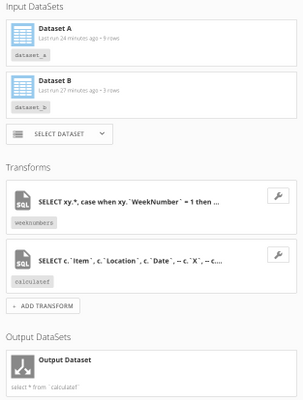Calculating a value for current week and rolling that value for subsequent weeks

Hi,

I have the following 2 datasets:

Dataset A

Item  Location   Date                  X    Y
A USA Sep 10(Current Week) 10 10
A USA Sep 17 15 20
A USA Sep 24 10 5
A Canada Sep 10(Current Week) 0 4
A Canada Sep 17 6 0
A Canada Sep 24 13 15
B USA Sep 10(Current Week) 10 50
B USA Sep 17 5 15
B USA Sep 24 15 20

Dataset B:

Item    Location     Z
A USA 15
B USA 25

I need an output dataset as follows:

O/P

Item   Location   Date   Final_Column
A USA Sep 10 15
A USA Sep 17 20
A USA Sep 24 15
B USA Sep 10 65
B USA Sep 17 75
B USA Sep 24 80

The calculation is as follows:

For current week, Final Column = (Z - X) + Y ----> 1st week value

For 2nd week, Final Column = (1st week value - X) + Y ----> 2nd week value

For 3rd week, Final Column = (2nd week value - X) + Y ----> 3rd week value

and so on ...

Additionally, if value of Final Column < 0 then Final Column = 0.

How to achieve this calculation in MySQL?

• Hi,

I came up with this

select a.item, a.location, a.date,
@value = case when @item <> a.item or @location <> a.location then b.z - a.x + a.y
else @value - a.x + a.y end final_column
from a inner join b on a.item = b.item and a.location = b.location,
(select @value := 0, @item := '', @location := '') x
order by a.item, a.location, a.date

I am getting the correct values except for when the value of final column becomes less than 0.

When the final_column value goes less than 0 , it should be hard-coded to 0.

How to achieve this in the code above?

Thanks.

• Hi, Prajju,

This is an interresting challenge! The part that makes it difficult is that, at each recursive step in the calculation, the final ouput must not be less than 0 (set to 0 if less than 0).

I did this in a SQL dataflow using two transforms. The first transform assigns a week order number, which is simply a row number partitioned by location and item and ordered by date. The second dataflow uses that week number as an input and calculates the final output value. I suppose it might be possible to combine those two steps into one, but this makes sense in my mind. Screen shot below.

The first transform:

SELECT
xy.*,
case when xy.`WeekNumber` = 1 then b.Z else 0 end as Z -- Z is only important in the week 1 calculation
from (
SELECT
a.Date,
a.X,
a.Y,
case
when @PrevLocation = a.`Location` and @PrevItem = a.`Item` then @WeekNumber := @WeekNumber + 1 -- if the current record has the same location and item as the previous record, then @WeekNumber = @WeekNumber + 1; otherwise, this must be week 1
else @WeekNumber := 1
end as WeekNumber,
@PrevLocation := a.`Location` as Location,
@PrevItem := a.`Item` as Item
from
`dataset_a` as a,
(select @WeekNumber := 0, @PrevLocation := '', @PrevItem := '') as init -- The From statement of the query is read first, so initialize variables here
order by a.`Location`,a.`Item`,a.`Date` -- since the variables are evaluated in the order in which they appear in the select statement, ordering is important
) as xy
left join `dataset_b` as b
on b.`Item` = xy.`Item`
and b.`Location` = xy.`Location`

The second transform:

SELECT
c.`Item`,
c.`Location`,
c.`Date`,
case
when c.`WeekNumber` = 1 then @F := c.`Z` - c.`X` + c.`Y` -- if the current record is week 1, the final value F = Z - X + Y
when c.`WeekNumber` > 1 and @F - c.`X` + c.`Y` > 0 then @F := @F - c.`X` + c.`Y` -- if the current record is week 2 or later (and if the previous value of F - current X + current Y > 0), final value is calculated recursively: F = F - X + Y
when c.`WeekNumber` > 1 and @F - c.`X` + c.`Y` <= 0 then @F := 0 -- if the current record is week 2 or later (and if the previous value of F - current X + current Y <= 0), final value is F = 0
end as final_column
from `weeknumbers` as c,
(select @F := 0) as init -- initialize the variable F
order by c.`Item`,c.`Location` desc,c.`WeekNumber`,c.`Date` -- again, since F is calculated incrementally, order matters

Hope this works for you.• Hi Dan,

I actually came up with a relatively easier solution:

select a.item, a.location, a.date,
@value = case when @item <> a.item or @location <> a.location then (ABS(b.z - a.x + a.y) + (b.z - a.x + a.y))/2
else (ABS(@value - a.x + a.y) + (@value - a.x + a.y))/2 end final_column
from a inner join b on a.item = b.item and a.location = b.location, (select @value := 0, @item := '', @location := '') x
order by a.item, a.location, a.date

So basically, if a value is negative, code it to 0. For example, if value = -15: (ABS(-15) + (-15)) / 2 --> (15 - 15)/2 --->  0

If positive retain the value . If value = 15 : (ABS(15) + 15)/2 ---> 15

Thanks,

Prajwal